Module 24 - Vectors

Introduction | Lesson 1 | Lesson 2 | Lesson 3 | Self-Test

Lesson 24.1: Vector Arithmetic

In this lesson you will learn to define vectors on the TI-89 and to perform three types of vector multiplication. Unit vectors will be discussed and two formats used to denote vectors will be identified.

Defining Vectors

Quantities that have both magnitude and direction are called vectors and are often represented by directed line segments, as illustrated below.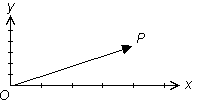The vector shown has an initial point at O and a terminal point at P.

Representing Vectors using Brackets

Vectors can be represented on the TI-89 by giving the coordinates of the tip of the arrow. For example, a vector that goes from the origin to the point (3, 2) is represented on the TI-89 with the notation [3, 2]. Note the use of brackets instead of parentheses to denote that the quantity is a vector.

• Perform NewProb
• Store the vector [3, 2] in the variable a on your TI-89
• The brackets are above the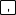and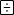keys.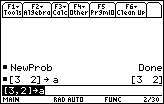You need to type in the "comma" between the components when entering a vector on the TI-89, but the calculator doesn't display it when it displays the vectors. This fact is illustrated in the above screen shot.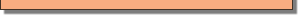Defining Unit Vectors i and j

The vector i is one unit long and points along the positive x-axis and the vector j is one unit long and points along the positive y-axis. Because the vectors i and j are each one unit long they are called unit vectors. Both i and j are shown below along with the vector [3, 2].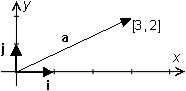Representing Vectors using i and j

Another notation uses the unit vectors i and j to represent a vector. The vector [3, 2] can also be written as

a = 3i + 2j

Notice that a, i, and j are written in bold to signify that they are vectors.

Finding the Length of a Vector

The length or magnitude of any vector a = [x, y] is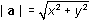The length of a = [3, 2] is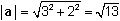units.

Vector Multiplication

There are three types of multiplication that involve vectors. Two types produce a vector and the remaining type produces a real number. Each type of multiplication is discussed below.

Scalar Multiplication of Vectors

Letting c represent a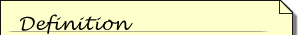A scalar is a real number. It has magnitude but no direction.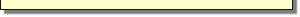scalar and a represent a vector, the scalar multiple ca is a vector whose length is |c| times that of the length of a and whose direction is the same as a if c is positive and the opposite direction from a if c is negative.

The coordinates of ca are found by multiplying each coordinate of a by c.

ca = c[a1, a2] = [ca1, ca2]

• Store the vector [3, -1] in the variable a on your calculator and multiply the vector by the scalar 2.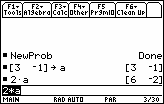• Multiply the vector [2, -5] by the scalar 3 without storing the vector to a variable.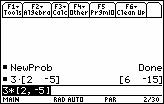Using the unitV Command

The vector that points in the same direction as a and has a magnitude of one can be found with the unitV command.

Determine a unit vector that points in the same direction as a = [3, 2].

• Open the Math menu by pressing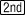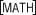• Open the Matrix submenu by selecting 4:Matrix and pressing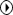• Scroll to L:Vector ops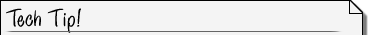Pressingwhile at the top of a menu displays the bottom of the menu, which can be faster than scrolling down to the bottom of the menu.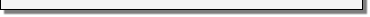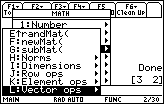• Open the vector operation's sub menu by pressingThe menu item "1:unitV(" should be highlighted.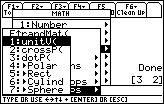• Pressto paste it to the Edit Line
• Enter unitV(a)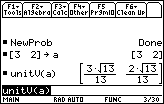Each component of a has been multiplied by the reciprocal of the magnitude of a to create the unit vector that points in the same direction as a. Note that the fractions have beenRationalizing (a denominator): A fraction is rationalized by writing it as an equivalent fraction with no radicals in the denominator.rationalized.

Finding Dot Products of Vectors

The second type of multiplication is called a dot product. The dot product of the two vectors [a1, a2] and [b1, b2] is defined to be a1 · b1 + a2 · b2.
The dot product of a and b can be found by using the command dotP, which is in the Math Matrix :Vector ops menu.

• Store the vector -2i + 5j into bCompute the dot product a · b.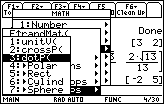• Paste dotP( to the Edit Line
• Enter dotP(a,b)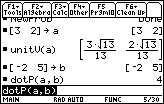The dot product a · b is 4.

Notice that the result of the dot product of two vectors is a real number, not a vector. The dot product is the same as the product of the magnitude of a, the magnitude of b and the cosine of the angle between a and b.
If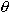is the angle between a and b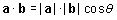Dot products are widely used in physics. For example, they are used to calculate the work done by a force acting on an object.

Projecting One Vector onto Another Vector

A projection can be thought of as the shadow of one vector on another. When the two vectors have the same initial point, the projection of b onto a is parallel to a and has the length of the shadow of b. The diagram below illustrates the projection of b onto a, written as projab and shown as the darker vector.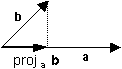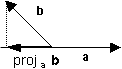Projections and Dot Products

The magnitude of the projection of b onto a, |projab|, is also called the component of b along a, and it is equal to | b | cos. Note that the component of b along a is equal to a · b / |a|.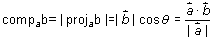Finding the Formula for Dot Products on your Calculator

The formula for finding the dot product of two vectors [a1, a2] and [b1, b2] can be derived on the TI-89.

• Enter dotP([a1,a2],[b1,b2])

24.1.1 Write the formula for finding the dot product of two vectors. Click here for the answer.

Defining Cross Products

The third type of multiplication is called a cross product and it is used in geometry and many situations in physics and engineering. The cross product a x b of two three-dimensional vectors is a vector that is perpendicular to both a and b. Ifis the angle between a and b, then the length of a x b is given by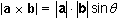The cross product is only defined for 3-dimensional vectors, but the TI-89 computes the cross product of 2-dimensional vectors by treating them as 3-dimensional vectors with 0 as the third component.

Finding Cross Products

A cross product can be calculated with the crossP command, which is found in the Math Matrix Vector ops menu.

Find the cross product a x b of the previously defined vectors a = [3, 2] and b = [-2, 5].

• Enter crossP(a,b)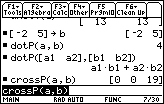[3, 2, 0] x [-2, 5, 0] = [0, 0, 19] = 0i + 0j + 19k

The result of a cross product is a vector that has three components, which means that it is a three-dimensional vector. The unit vector k points along the positive z-axis. The vector 0i + 0j + 19k points upward along the positive z-axis and has a length of 19.

The cross product of two vectors a and b is always perpendicular to each of the two vectors. It has a magnitude that is equal to the magnitude of a multiplied by the magnitude of the component of b that is perpendicular to a.

24.1.2 Find the cross product of the three-dimensional vectors a = 2i + j - 4k and b = -i – 2j + k. Describe the relationship between a, b, and their cross product. Click here to check your answer.

< Back | Next >